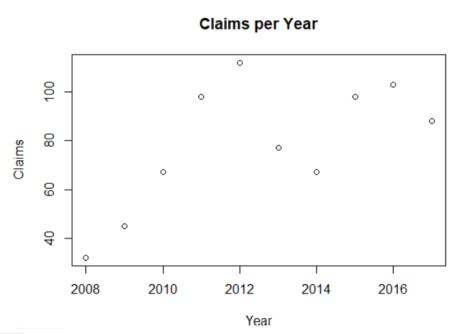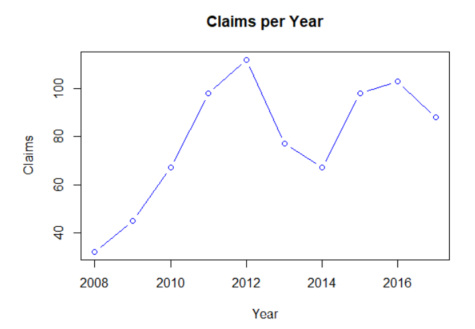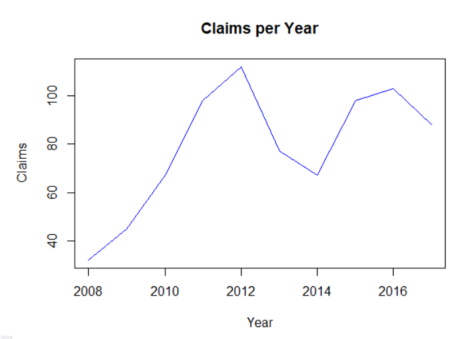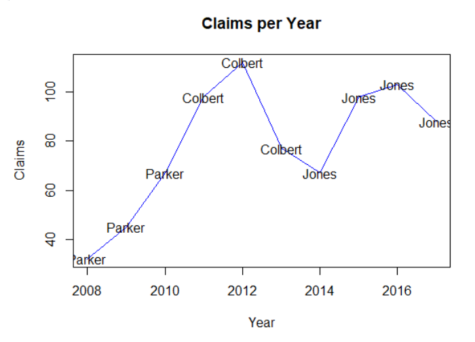# Plotting Data

R is also great for data visualization. Here are a few simple graphs.

```# Let's create the following dataset of numbers of claims per year:

```
```year <- c(2008:2017)
```
```claims <- c(32,45,67,98,112,77,67,98,103,88)
```
```disputes <- data.frame(year, claims)
```
```print(disputes)
```
` ##`
Year Claim
1 2008 32
2 2009 45
3 2010 67
4 2011 98
5 2012 112
6 2013 77
7 2014 67
8 2015 98
9 2016 103
10 2017 88

```# We can visualize that data using the plot() function.

```
```plot(disputes)

```
```##```

The plot() function, like other functions, in R has default settings.

``` # Consult the R documentation to learn how the function can be further customized.

```
```?plot()
```

For example, it is possible to add axis and graph labels.

```plot(disputes\$year,disputes\$claims, type = "p", xlab="Year", ylab="Claims",
main = "Claims per Year")

```
```##```

We can also plot data as both points and lines (“b”) and change the color of the graph.

```plot(disputes\$year,disputes\$claims, type = "b", col="blue", xlab="Year", ylab="Claims",
main = "Claims per Year")

```
```##```

We can even plot text labels in R using the function text() in combination with plot(). For instance, here we imagine that there were 3 different judges over our timeframe, which in turn had an impact on the data.

```judges <- c("Parker","Parker","Parker","Colbert","Colbert","Colbert","Jones","Jones","Jones","Jones")
```
```# Let's add the judge data to our dataframe and print the data again.

```
```disputes <- cbind(disputes, judges)
```
```print(disputes)
```
` ##`
Year Claim Judges
1 2008 32 Parker
2 2009 45 Parker
3 2010 67 Parker
4 2011 98 Colbert
5 2012 112 Colbert
6 2013 77 Colbert
7 2014 67 Jones
8 2015 98 Jones
9 2016 103 Jones
10 2017 88 Jones

```# We can add the name of the judges to the plot with the text() function.

```
```plot(disputes\$year,disputes\$claims, type = "l", col="blue", xlab="Year", ylab="Claims",
main = "Claims per Year")
```
``````
```text(disputes\$year,disputes\$claims, label=disputes\$judges)
```
``````

access_time Last update May 8, 2020.